Name:    Chapter 4 Test

Multiple Choice
Identify the choice that best completes the statement or answers the question.

Write the percent as a fraction or mixed number in simplest form.

1.

0.7%
 a.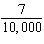c.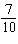b.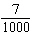d.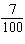2.

So far, 22% of the flights you make with your remote control robotic flying toy stay in the air for longer than one minute. What fraction of flights is this?
 a.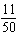c.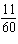b.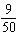d.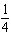Write the percent as a decimal.

3.

4%
 a. 0.4 c. 0.04 b. 0.004 d. 4

4.

11.9%
 a. 0.119 c. 11.9 b. 0.0119 d. 1.19

5.

0.68%
 a. 0.068 c. 68 b. 0.68 d. 0.0068

Tell which number is greater.

6.

80%, 0.08
 a. They are equal. b. 80% c. 0.08

7.

670%, 0.67
 a. 0.67 b. They are equal. c. 670%

Use a number line to order the numbers from least to greatest.

8.

1.54,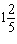, 15.8%, 1.6, 174%
 a., 1.54, 174%, 1.6, 15.8% c. 15.8%,, 1.54, 1.6, 174% b. 15.8%,, 1.54, 174%, 1.6 d., 1.54, 1.6, 174%, 15.8%

Find the percent of the number.

9.

20% of 70
 a. 14 c. 56 b. 10 d. 1400

10.

58% of 91
 a. 56.61 c. 38.22 b. 52.78 d. 50.54

11.

0.6% of 30
 a. 0.018 c. 18 b. 1.8 d. 0.18

Estimate the percent of the number.

12.

33% of 53
 a. 15 c. 30 b. 150 d. 7

Numeric Response

1.

How many minutes is 60% of 2 hours?

Round to a common percent.

2.

73%

1.

Can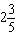be written as a percent? Explain.

Complete the statement using <, >, or =.

2.

160% of 8 _?_ 7.8% of 240

Other

1.

Extended Response  The percent of students that voted for a class trip is shown.
 Amusement Park Museum Circus 45% 10% 25%
a.   Write the percents as decimals.
b.   Write the percents as fractions.
c.   What percent of the students voted for a different class trip?